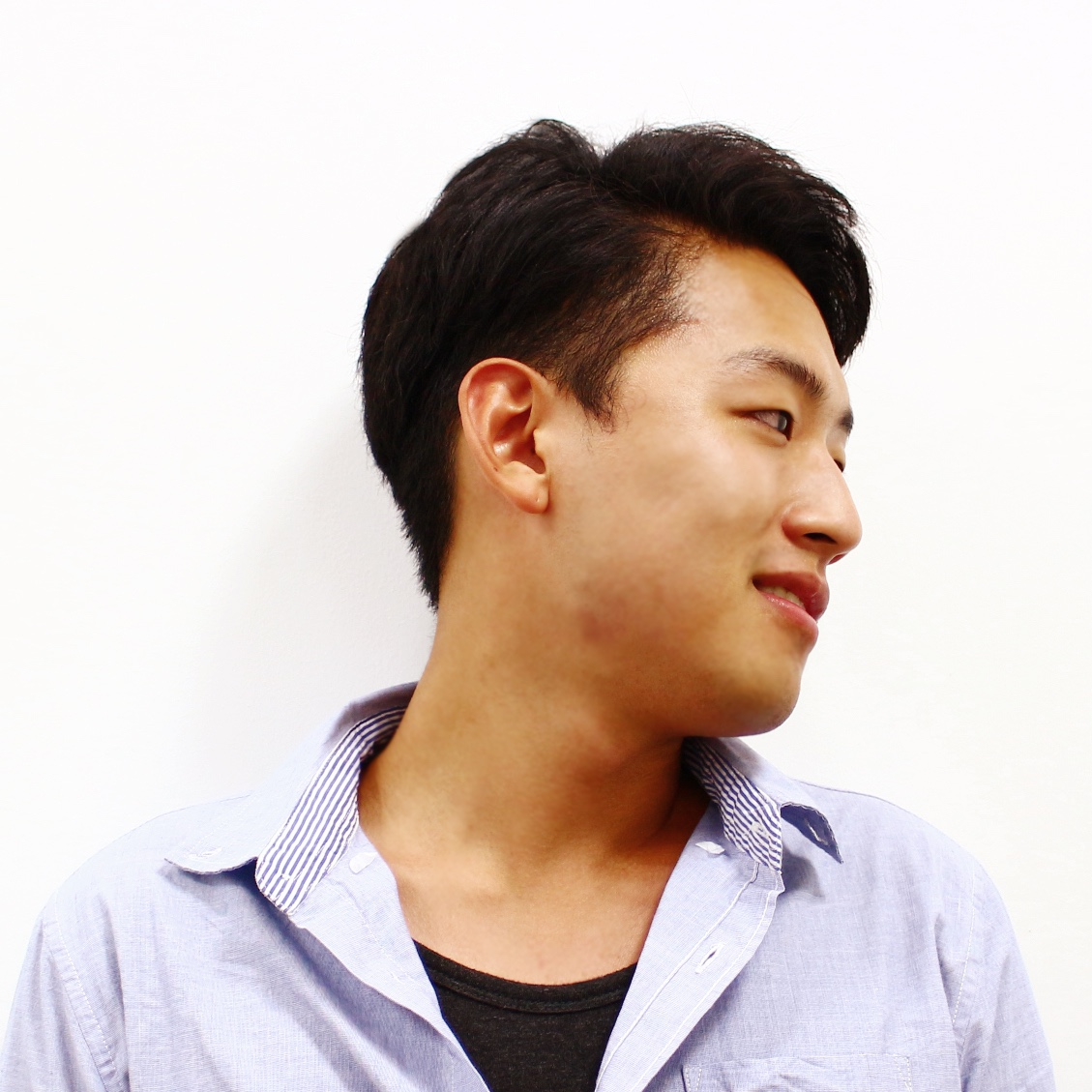/ Computer Vision

# 2. Unsupervised Cross-Domain Image Generation (DTN)

published to ICLR2017 by Yaniv Taigman, Adam Polyak, Lior Wolf

Learn $G: S \rightarrow T$ of two related domains, $S$ and $T$ without labels! (labels of images are usually expensive)## Baseline model$D$ : discriminator, $G$ : generator,
$f$ : context encoder. outputs feature. (128-dim)

\begin{equation}
R_{GAN} = \max_D \mathbb{E}_{x\sim\mathcal{D}_S} \log[1-D(G(x))] + \mathbb{E}_{x\sim\mathcal{D}_T} \log[D(x)]
\end{equation}

\begin{equation}
R_{CONST} = \mathbb{E}_{x\sim\mathcal{D}_S} d(f(x),f(G(x)))
\end{equation}

$f$-constancy : Does $x, G(x)$ have similar context?
$d$ : distance metric. ex) MSE
$f$ : "Pretrained" context encoder. Parameter fixed.
$f$ can be pretrained with classification task on $S$

Minimize two Risks : $R_{GAN}$ and $R_{CONST}$

Experimentally, Baseline model didn't produce desirable results.
Thus, similar but more elaborate architecture proposed

## Proposed "Domain Transfer Network (DTN)"First, $f$ : the context encoder now encode as $f(x)$ then $g$ will generate from it : $G = g(f(x))$

$g$ focuses to generate from given context $f(x)$

Second, for $x \in \mathbf{t}$, $x$ is also encoded by $f$ and applied $g$

"Pretrained $f$ on $S$" would not be good as much as on $T$. But enough for context encoding purpose
$L_{TID}$ : $G(x)$ should be similar to $x$
Also $D$ takes $G(x)$ and performs ternary (3-class) classification. (one real, two fakes)

## Losses

### Discriminator loss : $L_D$

\begin{equation}
L_D = -\mathbb{E}_{x \in \mathbf{s}} \log D_1 (G(x)) - \mathbb{E}_{x \in \mathbf{t}} \log D_2 (G(x)) - \mathbb{E}_{x \in \mathbf{t}} \log D_3 (x)
\end{equation}

$D_i(x): Probability$
$D_1(x)$ : generated from $S$? / $D_2(x)$ : generated from $T$? / $D_3(x)$ : sample from $T$?

### Generator : Adversarial Loss $L_{GANG}$

\begin{equation}
L_{GANG} = - \mathbb{E}_{x \in \mathbf{s}} \log D_3 (G(x)) - \mathbb{E}_{x \in \mathbf{t}} \log D_3(G(x))
\end{equation}

Fool $D$ to classify as sample from $T$

### Generator : $L_{CONST}$ and Identity preserving $L_{TID}$

\begin{equation}
L_{CONST} = \sum_{x \in \mathbf{s}} d(f(x),f(g(f(x)))
\end{equation}
, in feature level

\begin{equation}
L_{TID} = \sum_{x \in \mathbf{t}} d_2(x,G(x))
\end{equation}
, in pixel level

$d, d_2$ used as MSE in this work

\begin{equation}
L_{G} = L_{GANG} + \alpha L_{CONST}+ \beta L_{TID} + \gamma L_{TV}
\end{equation}

$L_{TV}$ is for output smoothing.

$L_G$ minimized over $g$
$L_D$ minimized over $D$

## Experiments

1. Street View House Numbers (SVHN) $\rightarrow$ MNIST
2. Face $\rightarrow$ Emoji
Both cases, $S$ and $T$ domains differ considerably
SVHN $\rightarrow$ MNIST
Pretrain $f$ on $SVHN_{f_TRAIN}$
Learn $G: SVHN_{DTN_TRAIN} \rightarrow MNIST_{TEST}$
Train a MNIST classifier on $MNIST_{TRAIN}$. will be used as evaluation purpose later
Domain transfer on $SVHN_{TEST}$ : $G(SVHN_{TEST})$### $f$

• 4 convs (each filters 64,128,256,128) / max pooling / ReLU
• input $32 \times 32$ RGB / output 128-dim vector.
• $f$ do not need to be very powerful classifier.
• achieves 4.95% error on SVHN test set
• Weaker in $T$ : 23.92% error on MNIST.
• Learn analogy of unlabeled examples

### $g$

• Inspired by DCGAN
• SVHN-trained $f$'s 128D representation $\rightarrow 32\times32$
• four blocks of deconv, BN, ReLU. TanH at final.
• $$L_{G} = L_{GANG} + \alpha L_{CONST}+ \beta L_{TID} + \gamma L_{TV}$$

$\alpha=\beta=15, \gamma=0$### Evaluate DTN

Train classifier on $MNIST_{TRAIN}$.
Architecture same as $f$
MNIST performance 99.4% test set.
Evaluate by testing MNIST classifier on $G(\mathbf{s}_{TEST}) = { G(x)|x \in \mathbf{s}_{TEST} }$ using $Y$ : $\mathbf{s}_{TEST}$ label.## Experiments: Unseen Digits

Study the ability of DTN to overcome omission of a class in samples.
For example, class '3' Ablation applied on

1. training DTN, domain $S$
2. training DTN, domain $T$
3. training $f$.

But '3' exists in testing DTN! Compare results.(a) The input images. (b) Results of our DTN. (c) 3 was not in SVNH. (d) 3 was not in MNIST. (e) 3 was not shown in both SVHN and MNIST. (f) The digit 3 was not shown in SVHN, MNIST and during the training of f.$S$ labeled, $T$ unlabeled, want to train classifier of $T$
Train k-NN classifier### Face $\rightarrow$Emoji

• face from Facescrub/CelebA
• emoji gained from bitmoji.com, not publicized
preprocess on emoji with heuristics. Align face.
• $f$ from DeepFace pretrained network. (Taigman et al. 2014) the author's previous work
• $f(x)$ is 256-dim
• $g$ outputs $64 \times 64$
• SR (Dong et al. 2015) to upscale final output.

### Results

choose $\alpha=100, \beta=1, \gamma=0.05$ via validationOriginal style transfer can't solve itDTN also can style transfer.
DTN is more general than Styler Transfer method.

## Limitations• $f$ usually can be trained in one domain, thus asymmetric.
• Handle two domains differently.
• $T \rightarrow S$ is bad.
• Bounded by $f$. Needs pre-trained context encoder.
• any better way to learn context without pretraining?
• Any more $S \rightarrow T$ tasks?

## Conclusion

• Demonstrate Domain Transfer, as an unsupervised method.
• Can be generalized to various $S \rightarrow T$ problems.
• $f$-constancy to maintain context of domain $S$ & $T$
• Simple domain adaptation and good performance
• inspiring work to future domain adaptation research

More open reviews at OpenReview.net#### Junho Cho

Integrated Ph.D course and Interested in Computer Vision, Deep Learning. For more information, tmmse.xyz/junhocho/